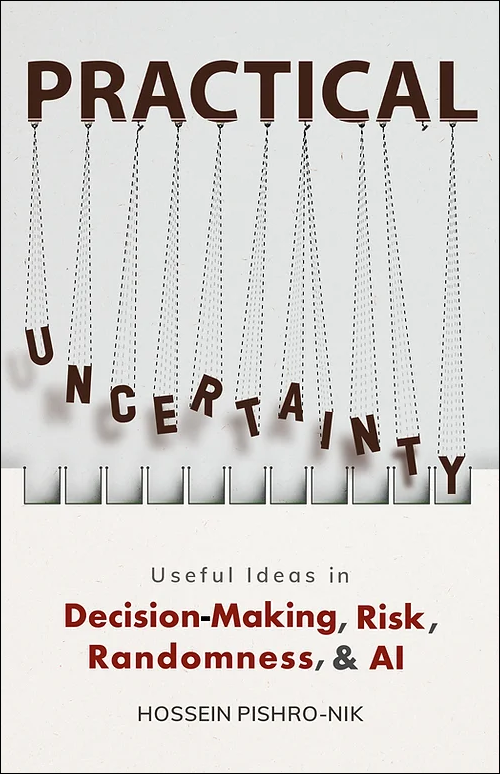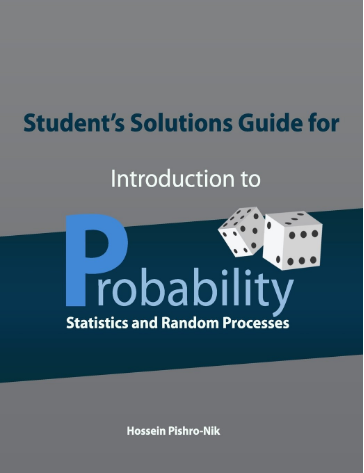## News:

 New book release: Practical uncertainty: Userful Ideas in Decision-Making, Risk, Randomness, & AI is now available on Amazon in both print and kindle format. Interested readers can visit our website to download a free sample.• A chapter on simulation using Python was added!

# Welcome

This site is the homepage of the textbook Introduction to Probability, Statistics, and Random Processes by Hossein Pishro-Nik. It is an open access peer-reviewed textbook intended for undergraduate as well as first-year graduate level courses on the subject. This probability textbook can be used by both students and practitioners in engineering, mathematics, finance, and other related fields.

The site includes:

• The entire textbook
• Short video lectures that aid in learning the material
• Online probability calculators for important functions and distributions
• A solutions manual for instructors
• Lecture slides

The print version of the book is available through Amazon here.# Book Coverage

This probability and statistics textbook covers:

• Basic concepts such as random experiments, probability axioms, conditional probability, and counting methods
• Single and multiple random variables (discrete, continuous, and mixed), as well as moment-generating functions, characteristic functions, random vectors, and inequalities
• Limit theorems and convergence
• Introduction to mathematical statistics, in particular, Bayesian and classical statistics
• Random processes including processing of random signals, Poisson processes, discrete-time and continuous-time Markov chains, and Brownian motion
• Simulation using MATLAB, R, and Python

# How to cite

You can cite this textbook as:

H. Pishro-Nik, "Introduction to probability, statistics, and random processes", available at https://www.probabilitycourse.com, Kappa Research LLC, 2014.

# Student’s Solutions Guide

Since the textbook's initial publication, many requested the distribution of solutions to the problems in the textbook. We published the student’s solutions guide which includes guided solutions to the odd-numbered end-of-chapter problems.

This guide is available on Amazon in both print and kindle format: